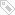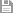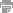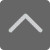TOP

高一物理：物理公式必备大全

2018-03-17 10:09:58 　来源：网络整理

高一物理：物理公式必备大全！同学们，学习物理重要,掌握学习物理的方法更重要。学好物理的“法宝”包括预习、听课、整理、应用(作业)、复习总结等。下面是小编特意为大家整理的高一物理：物理公式必备大全，供大家学习参考。

一, 质点的运动(1)----- 直线运动

1)匀变速直线运动

1.平均速度V平=S / t (定义式) 2.有用推论Vt 2 –V0 2=2as

3.中间时刻速度 Vt / 2= V平=(V t + V o) / 2

4.末速度V=Vo+at

5.中间位置速度Vs / 2=[(V_o2 + V_t2) / 2] 1/2

6.位移S= V平t=V o t + at2 / 2=V t / 2 t

7.加速度a=(V_t - V_o) / t 以V_o为正方向，a与V_o同向(加速)a>0;反向则a<0

8.实验用推论ΔS=aT2 ΔS为相邻连续相等时间(T)内位移之差

9.主要物理量及单位:初速(V_o):m/ s 加速度(a):m/ s2 末速度(Vt):m/ s

时间(t):秒(s) 位移(S):米(m) 路程:米

速度单位换算： 1m/ s=3.6Km/ h

注：(1)平均速度是矢量。(2)物体速度大,加速度不一定大。(3)a=(V_t - V_o)/ t只是量度式，不是决定式。(4)其它相关内容：质点/位移和路程/s--t图/v--t图/速度与速率/

2) 自由落体

1.初速度V_o =0 2.末速度V_t = g t

3.下落高度h=gt2 / 2(从V_o 位置向下计算)

4.推论V t2 = 2gh

注:(1)自由落体运动是初速度为零的匀加速直线运动，遵循匀变速度直线运动规律。

(2)a=g=9.8≈10m/s2 重力加速度在赤道附近较小,在高山处比平地小，方向竖直向下。

3) 竖直上抛

1.位移S=V_o t – gt 2 / 2 2.末速度V_t = V_o – g t (g=9.8≈10 m / s2 )

3.有用推论V_t 2 - V_o 2 = - 2 g S 4.上升最大高度H_max=V_o 2 / (2g) (抛出点算起)

5.往返时间t=2V_o / g (从抛出落回原位置的时间)

注:(1)全过程处理:是匀减速直线运动，以向上为正方向，加速度取负值。(2)分段处理：向上为匀减速运动，向下为自由落体运动，具有对称性。(3)上升与下落过程具有对称性,

如在同点速度等值反向等。

二 、 力(常见的力、力矩、力的合成与分解)

1)常见的力

1.重力G=mg方向竖直向下g=9.8 m/s2 ≈10 m/s2 作用点在重心 适用于地球表面附近

2.胡克定律F=kX 方向沿恢复形变方向 k：劲度系数(N/m) X：形变量(m)

3.滑动摩擦力f=μN 与物体相对运动方向相反 μ：摩擦因数 N：正压力(N)

4.静摩擦力0≤f静≤fm 与物体相对运动趋势方向相反 fm为最大静摩擦力

5.万有引力F=G m_1m_2 / r2 G=6.67×10-11 N·m2/kg2 方向在它们的连线上

6.静电力F=K Q_1Q_2 / r2 K=9.0×109 N·m2/C2 方向在它们的连线上

7.电场力F=Eq E：场强N/C q：电量C 正电荷受的电场力与场强方向相同

8.安培力F=B I L sinθ θ为B与L的夹角 当 L⊥B时: F=B I L ， B//L时: F=0

9.洛仑兹力f=q V B sinθ θ为B与V的夹角 当V⊥B时： f=q V B ， V//B时: f=0

注:(1)劲度系数K由弹簧自身决定(2)摩擦因数μ与压力大小及接触面积大小无关，由接触面材料特性与表面状况等决定。(3)fm略大于μN 一般视为fm≈μN (4)物理量符号及单位

B：磁感强度(T)， L：有效长度(m)， I:电流强度(A)，V：带电粒子速度(m/S), q:带电粒子(带电体)电量(C)，(5)安培力与洛仑兹力方向均用左手定则判定。

2)力矩

1.力矩M=FL L为对应的力的力臂，指力的作用线到转动轴(点)的垂直距离

2.转动平衡条件 M顺时针= M逆时针 M的单位为N·m 此处N·m≠J

三.平抛运动

1.水平方向速度V_x= V_o 2.竖直方向速度V_y=gt

3.水平方向位移S_x= V_o t 4.竖直方向位移S_y=gt2 / 2

5.运动时间t=(2S_y / g)1/2 (通常又表示为(2h/g) 1/2 )

6.合速度V_t=(V_x2+V_y2) 1/2=[ V_o2 + (gt)2 ] 1/2

合速度方向与水平夹角β: tgβ=V_y / V_x = gt / V_o

7.合位移S=(S_x2+ S_y2) 1/2 ,

位移方向与水平夹角α: tgα=S_y / S_x=gt / (2V_o)

注：(1)平抛运动是匀变速曲线运动，加速度为g，通常可看作是水平方向的匀速直线运动与竖直方向的自由落体运动的合成。(2)运动时间由下落高度h(S_y)决定与水平抛出速度无

关。(3)θ与β的关系为tgβ=2tgα 。(4)在平抛运动中时间t是解题关键。(5)曲线运动的物体必有加速度，当速度方向与所受合力(加速度)方向不在同一直线上时物体做曲

线运动。

2)匀速圆周运动

1.线速度V=s / t=2πR / T 2.角速度ω=Φ / t = 2π / T= 2πf

3.向心加速度a=V2 / R=ω2 R=(2π/T)2 R 4.向心力F心=mV2 / R=mω2 R=m(2π/ T)2 R

5.周期与频率T=1 / f 6.角速度与线速度的关系V=ωR

7.角速度与转速的关系ω=2πn (此处频率与转速意义相同)

周期(T)：秒(s) 转速(n)：r / s 半径(R):米(m) 线速度(V)：m / s

角速度(ω)：rad / s 向心加速度：m / s2

注：(1)向心力可以由具体某个力提供，也可以由合力提供，还可以由分力提供，方向始终与速度方向垂直。(2)做匀速度圆周运动的物体，其向心力等于合力，并且向心力只

改变速度的方向，不改变速度的大小，因此物体的动能保持不变，但动量不断改变。

3)万有引力

1.开普勒第三定律T2 / R3=K(4π2 / GM) R:轨道半径 T :周期 K:常量(与行星质量无关)

2.万有引力定律F=Gm_1m_2 / r2 G=6.67×10-11N·m2 / kg2方向在它们的连线上

3.天体上的重力和重力加速度GMm/R2=mg g=GM/R2 R:天体半径(m)

4.卫星绕行速度、角速度、周期 V=(GM/R)1/2

ω=(GM/R3)1/2 T=2π(R3/GM)1/2

5.第一(二、三)宇宙速度V_1=(g地

r地)1/2=7.9Km/s V_2=11.2Km/s V_3=16.7Km/s

6.地球同步卫星GMm / (R+h)2=m4π2 (R+h) / T2

h≈36000 km/h:距地球表面的高度

注:(1)天体运动所需的向心力由万有引力提供,F心=F万。(2)应用万有引力定律可估算天体的质量密度等。(3)地球同步卫星只能运行于赤道上空，运行周期和地球自转周期相同。

(4)卫星轨道半径变小时,势能变小、动能变大、速度变大、周期变小。(5)地球卫星的最大环绕速度和最小发射速度均为7.9Km/S。

以上就是小编为大家收集整理的高一物理：物理公式必备大全，希望能帮助到大家。同学们想要获得更多方面的辅导，可以拨打爱智康免费咨询电话：4000-121-121.那里有专业的老师为大家解答。标签：　高一物理公式必备大全保存　｜打印　｜关闭